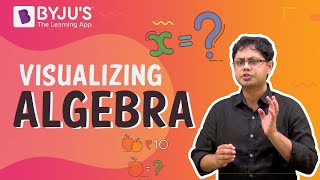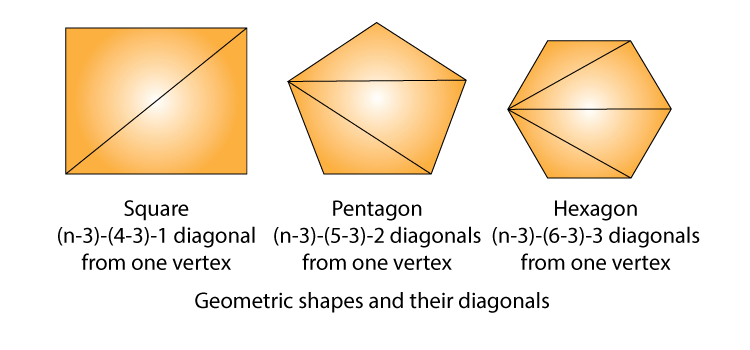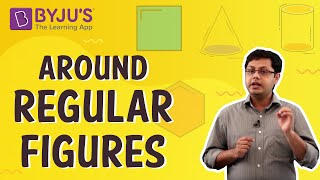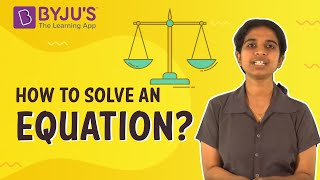# Algebra Class 6 Maths Notes - Chapter 11

Algebra is a branch of mathematics that can substitute letters for numbers to find the unknown. It can also be defined as putting real-life variables into equations and then solving them. The word Algebra is derived from Arabic “al-jabr”, which means the reunion of broken parts. Below are some algebra problems for students to practice.

## Introduction to Algebra

### Variable

A variable is an unknown quantity that is prone to change with the context of a situation.
Example: In the expression 2x+5, x is the variable.

### Constant

Constant is a quantity which has a fixed value. In the given example 2x+5, 5 is the constant.

### Terms of an Expression

Parts of an expression which are formed separately first and then added or subtracted, are known as terms.
In the above-given example, terms 2x and 5 are added to form the expression (2x+5).

### Factors of a term

Parts of an expression which are formed separately first and then added or subtracted, are known as terms.

• Factors of a term are quantities which cannot be further factorised.
• In the above-given example, factors of the term 2x are 2 and x.

### Coefficient of a term

The numerical factor of a term is called the coefficient of the term.
In the above-given example, 2 is the coefficient of the term 2x.
To know more about Term, factor and Coefficient, visit here.

## Like and Unlike Terms

### Like terms

Terms having the same variables are called like terms.>
Example: 8xy and 3xy are like terms.

### Unlike terms

Terms having different variables are called, unlike terms.
Example: 7xy and -3x are unlike terms.
To know more about “Algebra Basics”, visit here.

## Monomial, Binomial, Trinomial and Polynomial Terms

 Name Monomial Binomial Trinomial Polynomial No. of terms 1 2 3 >3 Example 7xy (4x−3) (3x+5y−6) (6x+5yx−3y+4)

To know more about Polynomials, visit here.

## Formation of Algebraic Expressions

Combinations of variables, constants and operators constitute an algebraic expression.

Example: 2x+3, 3y+4xy, etc.

To know more about Algebraic Expressions, visit here.

## Addition and Subtraction of Algebraic Expressions

### Addition and Subtraction of like terms

Sum of two or more like terms is a like term.

Its numerical coefficient will be equal to the sum of the numerical coefficients of all the like terms.
Example:  8y+7y=?

8y
+7y
___________
(8+7)y = 15y
____________

Difference between two like terms is a like term.

Its numerical coefficient will be equal to the difference between the numerical coefficients of the two like terms.
Example: 11z8z=?

11z

−8z
__________
(1-8)z = 3z
___________

### Addition and Subtraction of unlike terms

• For adding or subtracting two or more algebraic expressions, like terms of both the expressions are grouped together and unlike terms are retained as it is.
• Addition of 5x2+12xy and 7x2+xy+7x is shown below:

−5x2+12xy
7x2+xy+7x
__________
2x2+13xy+7x
__________

• Subtraction of 5x2+12xy and 7x2+xy+7x is shown below:

−5x2+12xy
−7x2+xy+7x
__________

12x2+11xy−7x
__________

To know more about Addition and Subtraction of Algebraic Expressions, visit here.

## Algebra as Patterns

#### For More Information On Algebra As A Pattern, Watch The Below Video.To know more about Algebra As a Pattern, visit here.

### Number patterns

• If a natural number is denoted by n, then its successor is (n + 1).
Example: Successor of n=10 is n+1=11.
• If a natural number is denoted by n, then 2n is an even number and (2n+1) is an odd number.
Example: If n=10, then 2n=20 is an even number and 2n+1=21 is an odd number.

#### For More Information On Number Patterns, Watch The Below Video.To know more about Number Pattern, visit here.

### Patterns in Geometry

• Some geometrical figures follow patterns which can be represented by algebraic expressions.
Example: Number of diagonals we can draw from one vertex of a polygon of n sides is (n – 3) which is an algebraic expression.Geometric shapes and their diagonals

## Algebraic expressions in perimeter  and area formulae

• Algebraic expressions can be used in formulating perimeter of figures.
Example: Let L be the length of one side then, the perimeter of :
1. An equilateral triangle = 3L.
2. A square = 4L.
3. A regular pentagon = 5L.
• Algebraic expressions can be used in formulating area of figures.
Example: Area of :
1. Square = l2 where l is the side length of the square.
2. Rectangle = l * b, where l and b are lengths and breadth of the rectangle.
3. Triangle = 1/2 b * h where b and h are base and height of the triangle.

#### For More Information On Perimeter And Area, Watch The Below Video.## What is the Equation?

An equation is a condition on a variable which is satisfied only for a definite value of the variable.

• The left-hand side(LHS) and right-hand side(RHS) of an equation are separated by an equality sign. Hence LHS = RHS.
• If LHS is not equal to RHS, then it is not an equation.

## Solving an Equation

Value of a variable in an equation which satisfies the equation is called its solution.

• One of the simplest methods of finding the solution of an equation is the trial and error method.

#### For More Information On Solving An Equation, Watch The Below Video.To know more about Linear Equations, visit here.
Other Important Links: